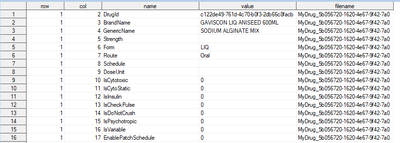## Data transpose

Is there a easier way to transpose a dataset from log to wide using proc sql that does not require sorting the dataset?

Can the transposed data automatically create numeric variables if all the values are such?
The example code is applied on the following dataset where some of the variables, such as "Isvariable" will only have numbers but they are appearing as character variables?
proc transpose data=tall out=wide(drop=_name_);
by filename row ;
id name;
var value;
run;2 REPLIES 2

## Re: Data transpose

Since value is of type character, all transposed variables will also be character.

Use a DATA step with SELECT:

``````data want;
set have;
by row;
retain
/* put your new variables here */
;
if first.row then call missing (/*all newvariables*/);
select (name);
......
when ('IsCytotoxic') iscytotoxic = input(value,1.);
......
end;
keep row /* all new variables */;
run;``````

## Re: Data transpose

It looks like your data is read from a number of infiles, row by row.

In that case, it may be (just guessing) that your data is grouped (but not sorted, because of the filename) already. Then it may be a possibility to use NOTSORTED:

``````proc transpose data=tall out=wide(drop=_name_);
by filename row notsorted;
id name;
var value;
run;``````

But your output variables will still be character, as the input variable is character.

If you need to convert the data to numeric, you can code the stuff in a datastep, as suggested by @Kurt_Bremser , although I would probably code it like this:

``````data wide;
do until(last.row);
set tall;
by filename row notsorted;
select(upcase(name));
...
when('ICYTOTOXIC') Icytotoxic=input(value,1.);
...
otherwise /* maybe write a warning in the log here */;
end;
end;
drop name;
run;``````
Discussion stats
• 2 replies
• 114 views
• 0 likes
• 3 in conversation